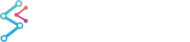﻿ OLD 3D Tutorial 04 - Changing the Axes Length and Chart Pitch and Yaw | JavaScript Chart DocumentationOLD 3D Tutorial 04 - Changing the Axes Length and Chart Pitch and Yaw

In the previous tutorial, it was hard to see the coordinates, because the axis range was much larger than the data range. So the points were small. And it was hard to understand where the coordinates are located, because they are in 3D.

So to address the first issue, we set the axis range close to the data range to make the points easier to see.

Specifically our data ranges from 1 to 6 we want the axis length to be, say, 0 and 6. So we set:

• com.scichart.data.model.DoubleRange = (Min = 1.0, Max = 5.0)
• com.scichart.data.model.DoubleRange = (Min = 0.0, Max = 6.0)

With:

Copy Code
```final NumericAxis3D xAxis = sciChart3DBuilder.newNumericAxis3D().withVisibleRange(0,6).withGrowBy(1, 1).build();
```

And to address the second issue, it's understand the position of the coordinates x,y,z in 3D space if we set one axis to zero. Below we have modified the code to create the points thus setting z to zero.

• (1,1,0)
• (2,2,0)
• (3,3,0)
• (4,4,0)
• (5,5,0)

Now you can more easily see that this is the graph of y = x, since we are looking at the xy plane from the side.If we set all three axes to (1,1,1) ... (5,5,5) we get the chart in the previous tutorial where the line appeared to be pointing straight out from the screen at a 45 degree angle.

At the bottom left as you rotate and title the screen with your fingers, meaning change the pitch and yaw. A graphic shows the orientation of the chart. It's small on the screen, so here it is enlarged:Below is the modified code. The default Yaw and Pitch are 45 so we use camera.setOrbitalYaw(54).

camera.setOrbitalYaw(54)
Copy Code
```protected void initExample() {
final Camera3D camera = sciChart3DBuilder.newCamera3D().build();
final XyzDataSeries3D<Double, Double, Double> dataSeries = new XyzDataSeries3D<>(Double.class, Double.class, Double.class);
for (int i = 1; i <= 5; i++) {
final double x = i;
final double y = i;
final double z = 0;
dataSeries.append(x, y, z);
}
final EllipsePointMarker3D pointMarker3D = sciChart3DBuilder.newEllipsePointMarker3D()
.withFill(ColorUtil.LimeGreen)
.withSize(2f)
.build();
final ScatterRenderableSeries3D rs = sciChart3DBuilder.newScatterSeries3D()
.withDataSeries(dataSeries)
.withPointMarker(pointMarker3D)
.withSeriesInfoProvider(new CustomSeriesInfo3DProvider())
.build();
surface3d.setCamera(camera);

final NumericAxis3D xAxis = sciChart3DBuilder.newNumericAxis3D().withVisibleRange(0,6).withGrowBy(1, 1).build();
final NumericAxis3D yAxis = sciChart3DBuilder.newNumericAxis3D().withVisibleRange(0,6).withGrowBy(1, 1).build();
final NumericAxis3D zAxis = sciChart3DBuilder.newNumericAxis3D().withVisibleRange(0,6).withGrowBy(1, 1).build();

surface3d.setXAxis(xAxis);
surface3d.setYAxis(yAxis);
surface3d.setZAxis(zAxis);
.withPinchZoomModifier3D().build()
.withZoomExtentsModifier3D().build());
camera.setOrbitalYaw(54);
camera.setOrbitalPitch(45);
```

## Pitch and Yaw

Since the chart is left-handed and the y axis it vertical rotation about the y axis (yaw) is in the counterclockwise direction. You could call this left. In terms of aviation, that makes sense as turning left and turning counterclockwise if the same thing. Since the x axis is horizontal rotation about the x axis (pitch) is counterclockwise. You could call this down. Again this makes sense when you think of an aircraft.

### Pitch Down

We started with this chart. This is oriented at the default yaw=45 and pitch=45.yaw=45 and pitch=45
Copy Code
```camera.setOrbitalYaw(45);
camera.setOrbitalPitch(90);
```

To make the chart tilt straight down, we change the pitch to 90 degrees. We change the yaw the same 45 degrees.This clearly looks like the chart has tiled straight down.

### Rotate Left

To turn left degrees we change yaw to -45 degrees, since rotation in the positive direction in a left-hand coordinate system is clockwise. So we want to go 90 degrees in the other direction.

Copy Code
```camera.setOrbitalYaw(-45);
camera.setOrbitalPitch(45);
```Look at the small graphic in the corner of the Android screen and you can see the new orientation of the xyz axes. The x axis has swung around 90 degrees: